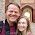## Tuesday, March 12, 2013

### Non-verbal Reasoning Test Construction - Part 1 Shape Creation

# R script

# I have been interested in developing a non-verbal reasoning test.  In the next few posts I will go through the code I used to generate a little over 300 items.

# First I defined a number of transformations which I will
flip = function(z) z*-1

# Two commands to flip the images horizontally or vertically
hflip = function(Z) cbind(flip(Z[,1]), Z[,2])
vflip = function(Z) cbind(Z[,1], flip(Z[,2]))

# Commands to rotate the images 90 degrees clockwise or counterclockwise
rotateCC = function(Z) cbind(Z[,2], flip(Z[,1]))
rotateC = function(Z) -1*cbind(Z[,2], flip(Z[,1]))

# A command to rotate the coordinates 180
rotate180 = function(Z) Z*-1# This command centers a vector so that the mean of all of the elements is zero 0
center = function(z) z-mean(c(min(z),max(z)))

# This function centers the matrix Z at zero for each column
Center = function(Z) cbind(center(Z[,1]),center(Z[,2]))
# This function scales and centers and object do that after centering the object the largest radius of any part of the object from the center is .5.
CenterScale = function(Z) Center(Z)/(2*max(abs(Center(Z))))

# This function makes the distance from any object from the 0,0 center to be unit 1 at the farthest point.
unitize = function(Z)  Z/max((Z[,1]^2+Z[,2]^2)^.5)

# This useful function samples i random draws without replacement from the vector V
rsample = function(V,i) V[order(runif(length(V)))][1:i]

# This function draws the coordinates for a polygon from the unit circle with number of sides equal to i.  If there is less than 3 sides specified then the number of sides is set to 50 making the polygon approximate a circle.
pgon = function(i, p=0, xscale=1) {
if (i<3 i="50</p">  cbind(sin(pi*2*(1:i+p)/i)*xscale, cos(pi*2*(1:i+p)/i))/2
}

# This function draws a star with the number of points equal to i.
star = function(i, p=0) {
pg1 = pgon(i,p)
pg2 = pgon(i,.5+p) * .25

pg = rbind(pg1[1,],pg2[1,])

for (j in 2:i) pg = rbind(pg, pg1[j,],pg2[j,])
pg
}

# This function draws a rectangle.  Speficy the rotation and it will construct the rectangle from around the unit circle.

# This function will draw a cross.  Specify the starting location in the same manner as the rectangle.
sreturn= rbind(c(C,S),c(-C,S),c(-C,C),c(-S,C),c(-S,-C),c(-C,-C),
c(-C,-S),c(C,-S),c(C,-C),c(S,-C),c(S,C),c(C,C))
unitize(sreturn)*.5
}

# Create a shape vector
shape_count=23
shape_vect = (1:23)[order(runif(shape_count))]
shape_vect = (1:23)

# Shape generating function
shape = function(shape_num=NA, sposition=c(0,0), size=1, dot=1, sdir=1) {
sreturn=c(0,0)
if (shape_num==shape_vect)  sreturn = pgon(1)
if (shape_num==shape_vect)  sreturn = pgon(3)
if (shape_num==shape_vect)  sreturn = pgon(4)
if (shape_num==shape_vect)  sreturn = pgon(4,xscale=3)
if (shape_num==shape_vect)  sreturn = pgon(4,.5)
if (shape_num==shape_vect)  sreturn = pgon(5)
if (shape_num==shape_vect)  sreturn = pgon(6,.5)
if (shape_num==shape_vect)  sreturn = pgon(7)
if (shape_num==shape_vect)  sreturn = pgon(8,.5)
if (shape_num==shape_vect) sreturn = star(3)
if (shape_num==shape_vect) sreturn = star(4,.5)
if (shape_num==shape_vect) sreturn = star(5)
if (shape_num==shape_vect) sreturn = star(6,.5)
if (shape_num==shape_vect) sreturn = rectangle(.2)
if (shape_num==shape_vect) sreturn = rectangle(1.8)
if (shape_num==shape_vect) sreturn = cross(.075)
if (shape_num==shape_vect) sreturn = cross(.4)
if (shape_num==shape_vect) sreturn = pgon(1,xscale=.5)
if (shape_num==shape_vect) sreturn = pgon(3,xscale=.3)
if (shape_num==shape_vect) sreturn = pgon(3,xscale=3)
if (shape_num==shape_vect) {
sreturn = pgon(1)[pgon(1)[,2]<.1,]
sreturn = cbind(sreturn[,1],sreturn[,2]+.3)
}

if (shape_num==shape_vect) {
sreturn = pgon(1)[pgon(1)[,1]<.1,]
sreturn = cbind(sreturn[,1]+.3,sreturn[,2])
}

if (shape_num==shape_vect) {
sreturn = pgon(1)[pgon(1)[,1]<0 p="">                        sreturn.reverse = sreturn[nrow(sreturn):1,]
sreturn.reverse[,1] = sreturn.reverse[,1]*.5
sreturn = rbind(sreturn,sreturn.reverse)
sreturn = cbind(sreturn[,1]+.3,sreturn[,2])
}
if (shape_num==shape_vect) {
sreturn = pgon(1)[(pgon(1)[,1]>=0)&(pgon(1)[,2]>=0),]
sreturn = rbind(sreturn,0)

sreturn = cbind(sreturn[,1]-.2,sreturn[,2]-.2)
}

sreturn = unitize(sreturn)*size
if (dot==1) sreturn = rbind(sreturn, cbind(NA,NA), size*(pgon(1)*.1+.75))

# Rotate
if (sdir==2)  sreturn=rotateCC(sreturn)
if (sdir==3)  sreturn=rotateC(sreturn)
if (sdir==4)  sreturn=rotate180(sreturn)

sreturn=cbind(sreturn[,1]+sposition,sreturn[,2]+sposition)

sreturn
}

# Plot a complete list of all of the shapes
par(mfrow=c(1,1))
plot(c(-1,1),c(-1,1), type="n")
for (i in 1:shape_count) {
polygon(shape(i, c(sin(i*2*pi/shape_count),cos(i*2*pi/shape_count))*.9,size=.11), col="red", border="black")
text(sin(i*2*pi/shape_count)*.9,cos(i*2*pi/shape_count)*.9,i,cex=2)
}
for (i in 1:shape_count) polygon(shape(i,size=.7))

# This is an example of the 23 shapes available to be generated from the above code.

# I hope someone finds it useful.  In the next post I will show how I used the generated shapes to generate a set of problems intended to provide a test for visual reasoning.

1.Hello,
I really liked your blog, I find it very interesting
In fact, I have a question, I want to use panel VAR in stata, what are the conditions that I must statisfy in order to do something like this:( unit root...)
and how to do it in stata?

Thank you very much

1.Hi Tunisian,

This is a good question and a bit too general for me to address. Each panel data method that you would like to use requires its own conditions or assumptions to be met. A typical assumption for a model that uses as an explanatory variable a lagged dependent variable is a stationarity assumption which is typically tested with a unit root type method. The general idea is that if your data is experiencing a random walk than including estimates of lagged variables will not be sufficient to give you a consistent estimator.

You can look at one of my previous posts for an implementation of this method: http://www.econometricsbysimulation.com/2012/06/he-randomly-walking-currency-market.html

Though I am definitely not very knowledgeable about time series procedures. Perhaps someone else has a better answer.

2.Hello,

Thank You for sharing this. Is it me or the second part of this tutorial is not jet published?

With Best Regards
Mait

1.Hi,

Sorry about that. The second part of the tutorial has not yet been published. I wanted to put some more work into it before but I will just put something out there.

Francis

3.Hi,

I read this post nearly 10 times but not able to understand, I google "non verbal reasoning shape construction" this key phrase and I am looking for the questions on shape construction. Can you plz guide me from where start so that I can easily understand your post.

1.4.